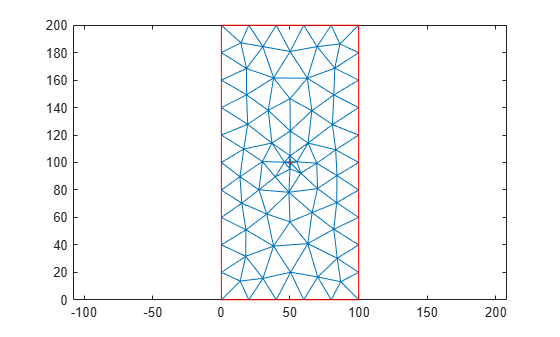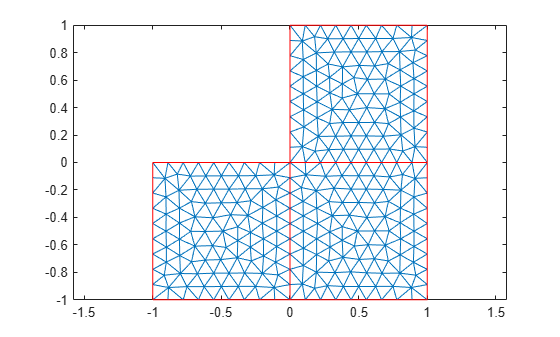meshQuality

Evaluate shape quality of mesh elements

Description

example

Q = meshQuality(mesh) returns a row vector of numbers from 0 through 1 representing shape quality of all elements of the mesh. Here, 1 corresponds to the optimal shape of the element.

example

Q = meshQuality(mesh,elemIDs) returns the shape quality of the specified elements.

example

Q = meshQuality(___,'aspect-ratio') determines the shape quality by using the ratio of minimal to maximal dimensions of an element. The quality values are numbers from 0 through 1, where 1 corresponds to the optimal shape of the element. Specify 'aspect-ratio' after any of the previous syntaxes.

Examples

collapse all

Evaluate the shape quality of the elements of a 3-D mesh.

Create a PDE model.

model = createpde;

Include and plot the following geometry.

importGeometry(model,'PlateSquareHoleSolid.stl');
pdegplot(model)Create and plot a coarse mesh.

mesh = generateMesh(model,'Hmax',35)
mesh =
FEMesh with properties:

Nodes: [3x487 double]
Elements: [10x213 double]
MaxElementSize: 35
MinElementSize: 17.5000

pdemesh(model)Evaluate the shape quality of all mesh elements. Display the first five values.

Q = meshQuality(mesh);
Q(1:5)
ans = 1×5

0.3079    0.2917    0.6189    0.6688    0.5571

Find the elements with the quality values less than 0.2.

elemIDs = find(Q < 0.2);

Highlight these elements in blue on the mesh plot.

pdemesh(mesh,'FaceAlpha',0.5)
hold on
pdemesh(mesh.Nodes,mesh.Elements(:,elemIDs), ...
'FaceColor','blue', ...
'EdgeColor','blue')Plot the element quality in a histogram.

figure
hist(Q)
xlabel('Element Shape Quality','fontweight','b')
ylabel('Number of Elements','fontweight','b')Find the worst quality value.

Qworst = min(Q)
Qworst = 0.1691

Find the corresponding element IDs.

elemIDs = find(Q==Qworst)
elemIDs = 1×2

10   136

Evaluate the shape quality of the elements of a 2-D mesh.

Create a PDE model.

model = createpde;

Include and plot the following geometry.

importGeometry(model,'PlateSquareHolePlanar.stl');
pdegplot(model)Create and plot a coarse mesh.

mesh = generateMesh(model,'Hmax',20)
mesh =
FEMesh with properties:

Nodes: [2x286 double]
Elements: [6x126 double]
MaxElementSize: 20
MinElementSize: 10

pdemesh(model)Find the IDs of the elements within a box enclosing the center of the plate.

elemIDs = findElements(mesh,'box',[25,75],[80,120]);

Evaluate the shape quality of these elements. Display the result as a column vector.

Q = meshQuality(mesh,elemIDs);
Q.'
ans = 12×1

0.2980
0.8253
0.2994
0.6581
0.7838
0.6104
0.3992
0.6921
0.2948
0.5726
⋮

Find the elements with the quality values less than 0.4.

elemIDs04 = elemIDs(Q < 0.4)
elemIDs04 = 1×4

9    19    69    83

Highlight these elements in green on the mesh plot. Zoom in to see the details.

pdemesh(mesh,'ElementLabels','on')
hold on
pdemesh(mesh.Nodes,mesh.Elements(:,elemIDs04),'EdgeColor','green')
zoom(10)Determine the shape quality of mesh elements by using the ratios of minimal to maximal dimensions.

Create a PDE model and include the L-shaped geometry.

model = createpde(1);
geometryFromEdges(model,@lshapeg);

Generate the default mesh for the geometry.

mesh = generateMesh(model);

View the mesh.

pdeplot(model)Evaluate the shape quality of mesh elements by using the minimal to maximal dimensions ratio. Display the first five values.

Q = meshQuality(mesh,'aspect-ratio');
Q(1:5)
ans = 1×5

0.8339    0.7655    0.7755    0.8301    0.8969

Evaluate the shape quality of mesh elements by using the default setting. Display the first five values.

Q = meshQuality(mesh);
Q(1:5)
ans = 1×5

0.9837    0.9605    0.9654    0.9829    0.9913

Input Arguments

collapse all

Mesh object, specified as the Mesh property of a PDEModel object or as the output of generateMesh.

Example: model.Mesh

Element IDs, specified as a positive integer or a matrix of positive integers.

Example: [10 68 81 97 113 130 136 164]

Output Arguments

collapse all

Shape quality of mesh elements, returned as a row vector of numbers from 0 through 1. The value 0 corresponds to a deflated element with zero area or volume. The value 1 corresponds to an element of optimal shape.

Example: [0.9150 0.7787 0.9417 0.2744 0.9843 0.9181]

Data Types: double

 Knupp, Patrick M. "Matrix Norms & the Condition Number: A General Framework to Improve Mesh Quality via Node-Movement." In Proceedings, 8th International Meshing Roundtable. Lake Tahoe, CA, October 1999: 13-22.

Partial Differential Equation Toolbox DocumentationGet trial now